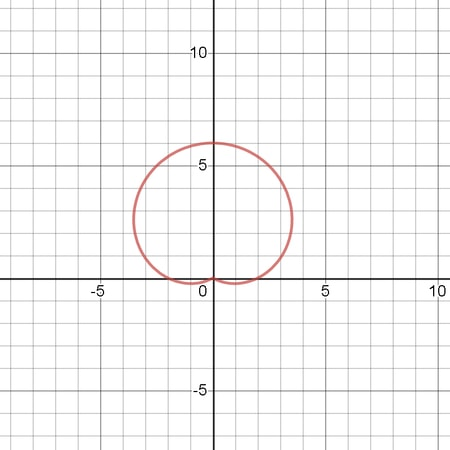# Sketch the polar curve r = 2 + 4\sin \theta

## Question:

Sketch the polar curve {eq}r = 2 + 4\sin \theta {/eq}

## Polar curve:

In such questions, we can see that the given equation cannot give a graphical image, so we convert the polar equation in cartesian form and then try to draw graph in cartesian axes.

## Answer and Explanation:

We are given :

{eq}r = 2 + 4\sin \theta {/eq}

Now substituting,

{eq}\displaystyle r = \sqrt{x^2+y^2} \displaystyle \\ \sin \theta = \frac{y}{\sqrt{x^2+y^2}} {/eq}

We get :

{eq}\displaystyle \sqrt{x^2+y^2} = 2 + 4 \frac{y}{\sqrt{x^2+y^2}} {/eq}

Now we can graph this as follows :#### Learn more about this topic:Graphs: Types, Examples & Functions

from High School Algebra II: Help and Review

Chapter 16 / Lesson 11
353K